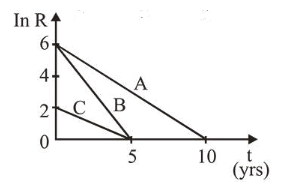# Acitvities of three radioactive substances A, B and C are represented by the curves A, B and C, in the figure.

Question:

Acitvities of three radioactive substances A, B and C are represented by the curves $A, B$ and $C$, in the figure. Then

their half-lives $\mathrm{T}_{\frac{1}{2}}(\mathrm{~A}): \mathrm{T}_{\frac{1}{2}}(\mathrm{~B}): \mathrm{T}_{\frac{1}{2}}(\mathrm{C})$ are in the ratio:1. (1) $2: 1: 1$

2. (2) $3: 2: 1$

3. (3) $2: 1: 3$

4. (4) $4: 3: 1$

Correct Option: , 3

Solution:

(3) Since, $R=R_{0} e^{-\lambda t}$

$\ln R=\ln R_{0}+(-\lambda \ln t)$

$\lambda=\frac{\ln 2}{t_{1 / 2}}=$ Slope

$\lambda_{A}=\frac{6}{10} \Rightarrow T_{A}=\frac{10}{6} \ln 2$

$\lambda_{B}=\frac{6}{5} \Rightarrow T_{B}=\frac{5 \ln 2}{6}$

$\lambda_{C}=\frac{2}{5} \Rightarrow T_{C}=\frac{5 \ln 2}{6}$

$\therefore T_{\frac{1}{2} A}: T_{\frac{1}{2} B}: T_{\frac{1}{2} C}=\frac{10}{6}: \frac{5}{6}: \frac{15}{6}=2: 1: 3$